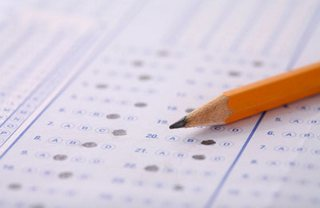In this one PDF, MCQs of all chapters of FSc Part1 are given. There are seven chapters. Answers of MCQs is starting from page 71.

• $i^{13}=$………….
• (A) $i$
• (B) 1
• (C) -1
• (D) 2
• Set of all possible subsets of $S$ is called
• (A) Equivalent sets
• (B) Empty set
• (C) Power set
• (D) subset
• Cube root of unity are
• (A) $1, \omega, \omega^2$
• (B) $-1, \omega, \omega^2$
• (C) $-1, -\omega, -\omega^2$
• (D) $1, -1, 2$
• The equation $ax^2+bx+c=0$ will be quadratic if
• (A) $a=0, b\neq 0$
• (B) $a\neq 0$
• (C) $a=b=0$
• (D) $b=$ any real number
• An open sentence formed by using a sign of '=' is a/an
• (A) equation
• (B) formula
• (C) rational fraction
• (D) theorem
• An arrangement of the number according to some definite rule is called
• (A) sequence
• (B) combination
• (C) series
• (D) permutation
• The equation $ax^2+bx+c=0$ will be quadratic if
• (A) $a=0, b\neq 0$
• (B) $a\neq 0$
• (C) $a=b=0$
• (D) $b=$ any real number
• $n!=n(n-1)(n-2)...3\cdot 2\cdot 1$ defined only when $n$ is
• (A) positive integer
• (B) integer
• (C) real number
• (D) whole number
• If the angle of rotation is counter clockwise then angle is
• (A) negative
• (B) positive
• (C) non-negative
• (D) none of these